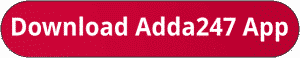Latest Banking jobs   »   IBPS po pre quant

# Quantitative Aptitude Quiz For IBPS PO Prelims 2023 -15th July

Directions (1-5): Bar graph given below show total number of students in five different schools (A, B, C, D & E) in two different years (2021, 2022). Read the following bar graph carefully and answer the questions given below.Q1. The ratio of number of boys to girls in school B and that in D in 2021 is 11:7 & 9:5 respectively. Find the difference between total number of boys in B & D together and number of total girls in both schools in 2021?
(a) 120
(b) 160
(c) 180
(d) 240
(e) 200
Q2. Find the total number of students in school A in 2022 is what percent (approximately) more or less than total number of students in school C in 2021?
(a) 14%
(b) 18%
(c) 26%
(d) 22%
(e) 8%
Q3. If total number of students in school F in 2022 is 66.66% more than total number of students in E in 2022, then find total number of students in school D and F together in 2022?
(a) 1180
(b) 1140
(c) 1120
(d) 1080
(e) 1160
Q4. Find the ratio of total number of students in E and that in C together in 2022 to total number of students in B and that in D in 2021?
(a) 5:2
(b) 3:7
(c) 3:5
(d) 4:7
(e) 3:2
Q5. Average number of students in A, B & C in 2022 is how much more or less than average number of students in D & E in 2021?
(a) 240
(b) 200
(c) 160
(d) 180
(e) 280

Directions (6-10):- What will come in place of the question mark (?) in the following questions?
Q6. 7.5× 8 – 10 = ? × 2.5
(a) 15
(b) 20
(c) 25
(d) 30
(e) 35

Q7. 7394+6295-3689 = ? × 40
(a) 320
(b) 240
(c) 280
(d) 250
(e) 300

Q8. 9×9÷ 3+9× 123 = ? – 19 -23
(a) 1176
(b) 1174
(c) 1177
(d) 1175
(e) 1178

Q9. 13× 23+ 27× 37 = (?) – 302
(a) 1620
(b) 1540
(c) 1700
(d) 1500
(e) 1600

Q10. 493+287-334 = -54 + ? × 5
(a) 90
(b) 100
(c) 110
(d) 95
(e) 85

Solutions. .                              .

## FAQs

### When will the IBPS PO prelims 2023 be conducted?

IBPS PO Prelims will be conducted on 23, 30 September, and 1 October 2023.

#### Congratulations!Union Budget 2023-24: Free PDF